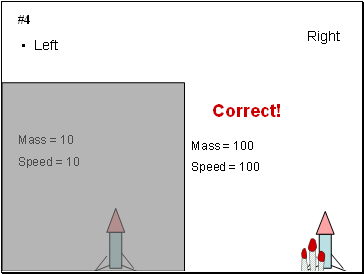# Altitude of a rocket- mass vs initial speedPage 1

#### WATCH ALL SLIDES

Slide 1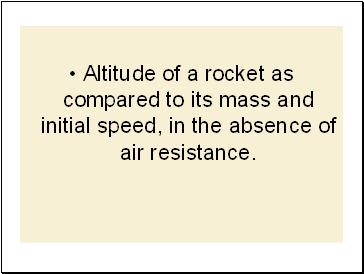Altitude of a rocket as compared to its mass and initial speed, in the absence of air resistance.

Slide 2Determine which rocket will go the highest. All rockets will be launched vertically from the ground.

Slide 3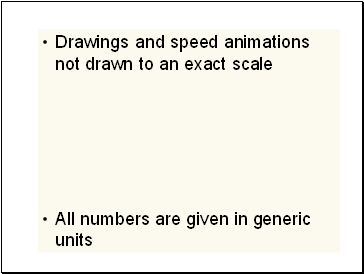Drawings and speed animations not drawn to an exact scale

All numbers are given in generic units

Slide 4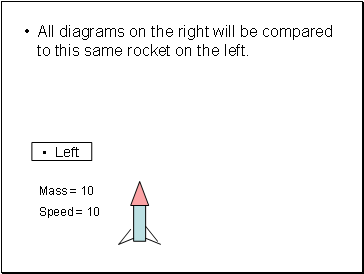All diagrams on the right will be compared to this same rocket on the left.

Mass = 10

Speed = 10

Left

Slide 5Raise your left hand if you think the diagram on the left will go the highest

Raise your right hand if you think the diagram on the right will go the highest

Slide 6#1

Slide 7Left

Right

#1

Mass = 10

Speed = 10

Mass = 10

Speed = 20

Slide 8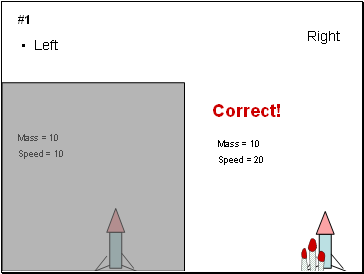Left

Right

#1

Mass = 10

Speed = 10

Mass = 10

Speed = 20

Correct!

Slide 9#2

Slide 10Left

Right

#2

Mass = 10

Speed = 10

Mass = 10

Speed = 1

Slide 11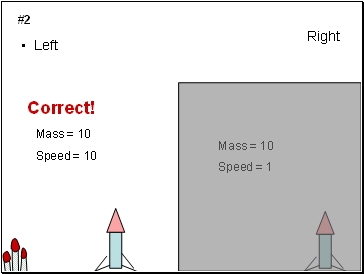Left

Right

#2

Mass = 10

Speed = 10

Mass = 10

Speed = 1

Correct!

Slide 12#3

Slide 13Left

Right

#3

Mass = 10

Speed = 10

Mass = 20

Speed = 10

Slide 14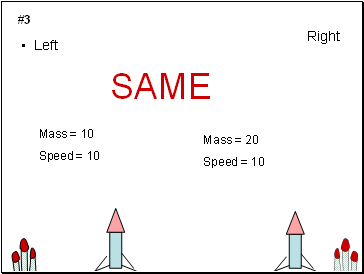Left

Right

#3

Mass = 10

Speed = 10

Mass = 20

Speed = 10

SAME

Slide 15#4

Slide 16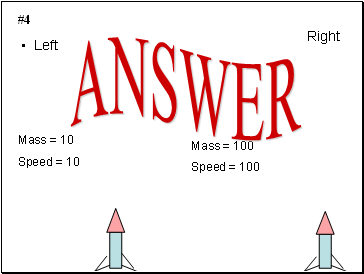Left

Right

#4

Mass = 10

Speed = 10

Mass = 100

Speed = 100